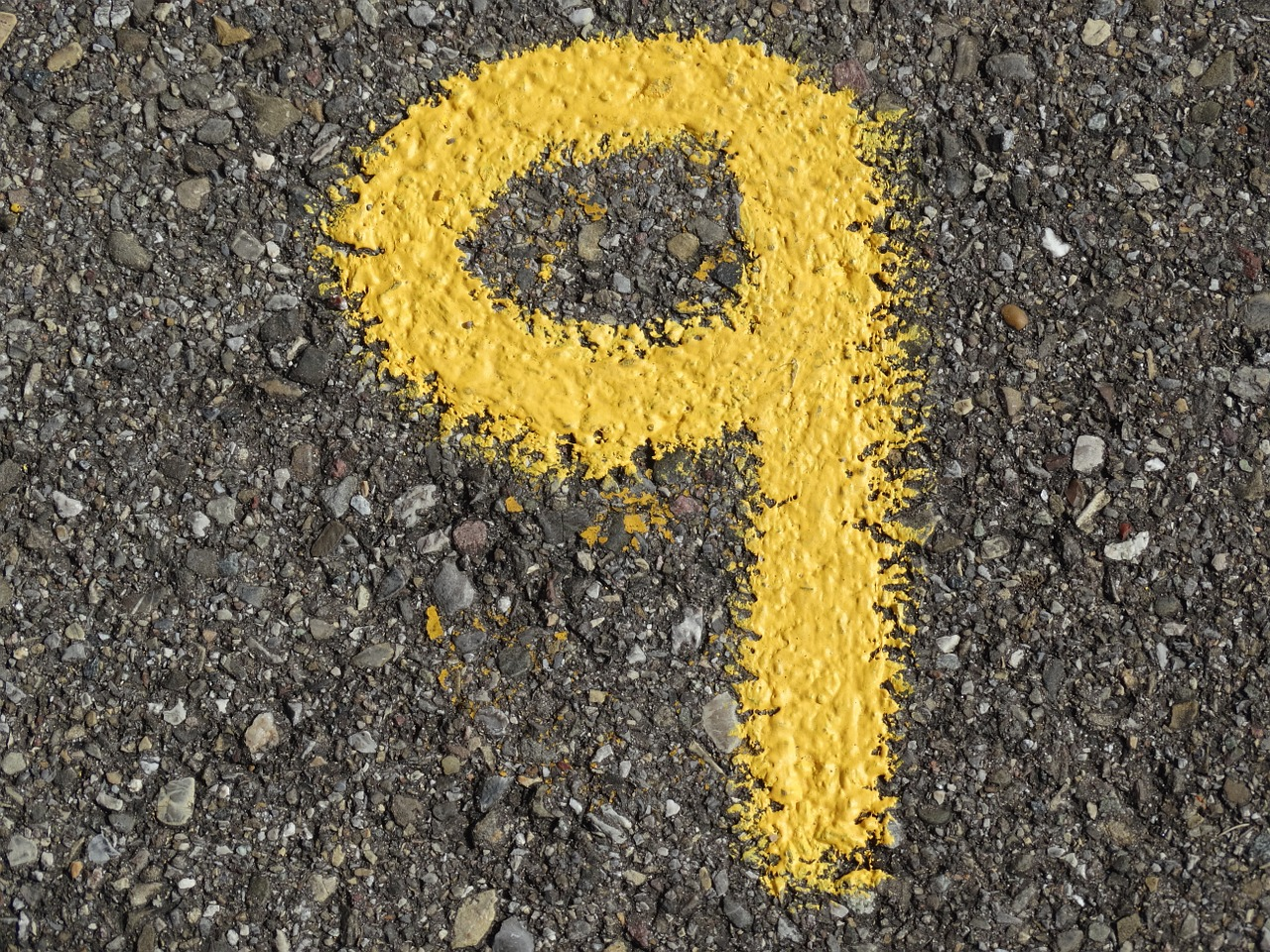Posted August 14, 2019

# The divisible by 9 error check tool

## Accountants, bookkeepers and tax specialists have a number of tools to check work and catch errors as they occur.

### Does the difference divide by 9?

When a balance doesn’t reconcile, one helpful tool in order to identify how the error has arisen, is to ask “does the difference divide by 9?” If it does, then we know that this is likely to be a transposition error. This is where a number has been recorded with two digits flipped.

###Try it yourself

Write down any list of 5 numbers: e.g.

£30
£59
£230
£98
£3

The sum of the above should be: £420, but if we accidentally flip (or transpose) any of the numbers, we will get a different total:

£03
£59
£230
£98
£3

The total here is £393, our mistake has led to a difference of £27- which is divisible by 9. Try flipping any other two digits an you will see that you will always get an answer different from £420, but that the difference is always divisible by 9.

### Save time search for errors

So, when we find a difference and it’s divisible by 9, it’s more likely than not that we have accidentally typed two numbers in the wrong way round, which saves time searching for other errors which could have made the difference.

### Why does the difference always divide by 9? Does it really apply to any 2 digits?

We can prove this mathematically and will attempt to do so below using high school or 6th form maths – but if you don’t have the head for mathematical proofs, then rest assured that it does always work.

### Here’s the Proof

Let us assume we have any two digits which are next to each other of any base 10 number i.e. any normal number. Those digits, a and b, are next to each other and so can be represented in the following way:

a10n b10(n-1)

This tells us that the first digit is “worth” 10 times more than the second digit. i.e. if you have a number 23, and you split them into 2 and 3, the two is actually representing 20, and the 3 just represents 3.

The digits you have chosen can thus be represented as:

ab = a10n + b10(n – 1)

Which can be factorised:

ab= 10(n−1)(a101+ b)

ab= 10(n−1)(10a+ b)

If the digits were switched around, we would instead have:

“ba”= 10(n−1)(10b+ a)

Let us find the difference between the two:

ab-“ba”= 10(n−1)(10a+b)−10(n−1)(10b+a)

ab-“ba”= 10(n−1)[(10a+b)−(10b+a)]

ab-“ba”= 10(n−1)(10a−10b+b−a)

ab-“ba” = 10(n−1)(9a-9b)

ab-“ba” = 9[10(n−1)(a-b)]

As you can see, once we simplify and factorise, the end result will always be a multiple of 9.